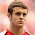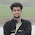## Monday, 26 August 2013

### Data Structure : Doubly Link List

The Doubly Linked List is a Java Singly Linked List with a reference present to refer to the previous node as well.
Doubly Linked List is use in scenarios where deletion is more frequent.

Below is the video that explains the insert mechanism in a double linked list.

Below is the structure of each node. Note unlike Singly Linked List, it has two nodes. Next and Prev
```class Node {
public int data;
public Node next;
public Node prev;
}
```
A 'start' node is used to always point to the first node. This makes sure the Linked List is not lost in memory.
```static Node start;
```
Below code is for addition of nodes in a Doubly Linked List. The code adds the element to the end of the list. Some modification can be made to add a element in between by passing the index along with data.
``` private static void add(int i) {
// Create new node
Node p = new Node();
p.data = i;
// Check if List is empty, If yes than point 'start' to the newly
// created node
if (start == null) {
start = p;
} else {
// If the list is not empty, than traverse till the end of the list
Node s = start;
while (s.next != null) {
s = s.next;
}
s.next = p;
p.prev = s;
}

}
```
Below code is for deletion of a node. The parameter passed is the value of int in each node. The values is matched against each node and than that node is deleted. It is important the the deleted node is made null so that it is available for garbage collection.
``` private static void delete(int i) {
System.out.println("Deleting element : " + i);
//Create a reference node
Node s = start;
//Iterate till the element to be deleted is found
while (s.data != i) {
s = s.next;
}
//Attach the previous element's 'next' to 'next' of the to be deleted element
s.prev.next = s.next;
//Attach the 'next' elements 'prev' to 'prev' of the to be deleted element
s.next.prev=s.prev;

//Make deleted element available for garbage collection
s.prev=null;
s.next=null;

}

```
Below is the complete code to test the Doubly Linked List
```package com.doubly;

class Node {
public int data;
public Node next;
public Node prev;
}

static Node start;

public static void main(String[] args) {
display();
System.out.println("\n3.Delete element");
delete(30);
display();

}

private static void add(int i) {
// Create new node
Node p = new Node();
p.data = i;
// Check if List is empty, If yes than point 'start' to the newly
// created node
if (start == null) {
start = p;
} else {
// If the list is not empty, than traverse till the end of the list
Node s = start;
while (s.next != null) {
s = s.next;
}
s.next = p;
p.prev = s;
}

}

private static void display() {

Node s = start;

while (s != null) {

System.out.println(s.data);
s = s.next;
}

}

private static void delete(int i) {
System.out.println("Deleting element : " + i);
//Create a reference node
Node s = start;
//Iterate till the element to be deleted is found
while (s.data != i) {
s = s.next;
}
//Attach the previous element's 'next' to 'next' of the to be deleted element
s.prev.next = s.next;
//Attach the 'next' elements 'prev' to 'prev' of the to be deleted element
s.next.prev=s.prev;

//Make deleted element available for garbage collection
s.prev=null;
s.next=null;

}

}

```
Output
```1.Adding elements

10
20
30
40

3.Delete element
Deleting element : 30

10
20
40
```
The advantage of double linked list over singly linked list is at the time of deleting a node from between the list. In singly linked list since there is no reference to the prev node, 2 round of iteration is to be done. First to get to the node which is to be deleted and then to get to the node just febore the node to be deleted.

In doubly linked list, by using the prev reference, deletion can be done in just one iteration.

Doubly Linked List are used in Many Collection classes such as LinkedList, HashMap, TreeMap, HashTable etc.

A example of usage of DoublyLinkedList in HashMap is provided Java Custom Hash Map : Internal Working.

1.Hi Hunaid,
The above code might not work for the first element deletion.The below code might work.

private static void delete(int i) {
Nodes s = start;
if(s.data == i){
start = s.next;
s.next.prev = null;
}else{
while (s.data != i) {
s = s.next;
}
s.prev.next=s.next;
}
}

1.Yes, you are right. I missed this scenario. Thank you.

2.Hi...I have gone through the below link which was very useful.
http://www.java-redefined.com/2013/08/java-collections-internal-working.html
Can you please cover HashTable as well? It would be great if you explain with more details with more real time scenarios.

3.I have a question. Looks like every time when we add a node to LinkedList, we traverse to the end and add the new element, instead if we had a variable that pointed to the 'last', we could just add the new node at the end and update the variable 'last'.

1.Thats a valid point Sokkalingam,
This approach will save the traversing required to get to the last node.

Thanks